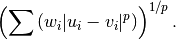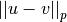# scipy.spatial.distance.wminkowski¶

scipy.spatial.distance.wminkowski(u, v, p, w)[source]

Computes the weighted Minkowski distance between two 1-D arrays.

The weighted Minkowski distance between u and v, defined asParameters : u : (N,) array_like Input array. v : (N,) array_like Input array. p : int The order of the norm of the difference. w : (N,) array_like The weight vector. wminkowski : double The weighted Minkowski distance between vectors u and v.

#### Previous topic

scipy.spatial.distance.sqeuclidean

#### Next topic

scipy.spatial.distance.yule